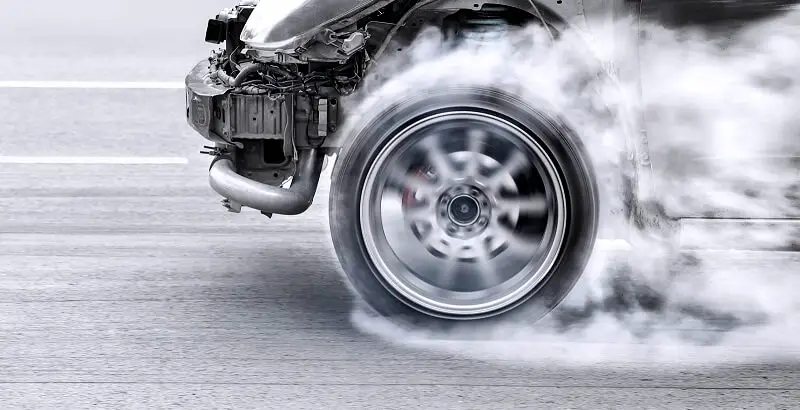# Understanding The Revolutions Per MileTires are essential to any vehicle, and understanding their performance characteristics is crucial for safety and efficiency. One such characteristic is revolutions per mile (RPM), a measure of the number of times a tire rotates as the vehicle travels one mile.

We will explore the significance of revolutions per mile in determining tire performance, how it is measured, the factors that affect it, and its importance in tire maintenance.

How Revolutions Per Mile is Measured?
Measuring revolutions per mile involves a combination of mathematical calculations and physical measurements. The process typically includes the following steps:

1. This can be done using a measuring tape or specialized equipment, such as a tire circumference gauge. The circumference is measured along the tire’s outer edge, following the tread path.
2. The number of revolutions per mile is obtained by dividing the tire’s circumference into the total distance of one mile (5,280 feet or 63,360 inches). The resulting value represents the number of times the tire will rotate to cover a distance of one mile.

For example, if a tire has a circumference of 80 inches, its RPM value would be RPM = 63,360 inches (1 mile) / 80 inches = 792 revolutions per mile.

Factors Affecting RPM
Various factors can impact a tire’s revolutions per mile, including tire size, type, and driving conditions. Some of these factors are:

• The tire’s overall diameter, width, and aspect ratio directly influence its RPM. Tires with larger diameters and lower aspect ratios tend to have lower RPM values, while smaller-diameter tires with higher aspect ratios typically have higher RPM values. Different tire types, such as high-performance tires, all-terrain tires, or winter tires, may have unique RPM characteristics due to differences in tread patterns and rubber compounds.
• A tire’s RPM can also be affected by vehicle load, inflation pressure, and driving style. For instance, an underinflated tire will have a smaller effective diameter, leading to a higher RPM value. Similarly, aggressive driving, high-speed cornering, and rapid acceleration or braking can cause changes in RPM, impacting tire performance and wear.

Tire Maintenance
Monitoring a tire’s revolutions per mile can provide valuable insights into its overall condition and help identify potential maintenance issues. Some key aspects of tire maintenance that can benefit from RPM monitoring are:

1. An increase in RPM over time may indicate excessive tire wear, uneven wear patterns, or other issues such as misalignment or imbalance. By regularly monitoring RPM values and comparing them to the manufacturer’s specifications, vehicle owners can identify potential problems and address them before they lead to more serious issues or accidents.
2. Understanding a tire’s RPM characteristics can help vehicle owners plan for routine maintenance tasks, such as tire rotations, balancing, and alignment checks. By keeping track of RPM values and comparing them with the manufacturer’s recommended maintenance intervals, vehicle owners can ensure that their tires are properly maintained and achieve optimal performance and longevity.

How To Calculate Rpm From Diameter?
To calculate revolutions per mile (RPM) from the diameter of a tire, follow these steps:

• Determine the tire’s diameter: Measure the diameter of the tire, which is the distance from one end of the tire to the other, passing through the center.
• Calculate the tire’s circumference: Use the formula for the circumference of a circle, which is:

C = π × D

Where C is the circumference, π (pi) is a mathematical constant approximately equal to 3.14159, and D is the diameter of the tire.

1. Convert the circumference to inches: If the circumference was measured in a different unit, such as centimeters or meters, convert it to inches using the appropriate conversion factors. For example, to convert from centimeters to inches, divide the value by 2.54.
2. Calculate revolutions per mile: Divide the total number of inches in one mile by the tire’s circumference (in inches) to determine the number of revolutions per mile. There are 63,360 inches in one mile, so the formula is:

RPM = 63,360 inches (1 mile) / C (in inches)

For example, if the tire’s diameter is 30 inches, the calculation would be:

C = π × D = 3.14159 × 30 inches = 94.25 inches

RPM = 63,360 inches / 94.25 inches = 672.47 revolutions per mile

In this example, the tire would make approximately 672.47 revolutions for every mile traveled.

Conclusion
Revolutions per mile are a crucial parameter for understanding and optimizing tire performance, as it directly impacts factors such as fuel efficiency, road noise, and handling. By measuring RPM and considering factors that can influence it, such as tire size, type, and driving conditions, vehicle owners can make informed decisions about tire selection and maintenance.

Scroll to Top